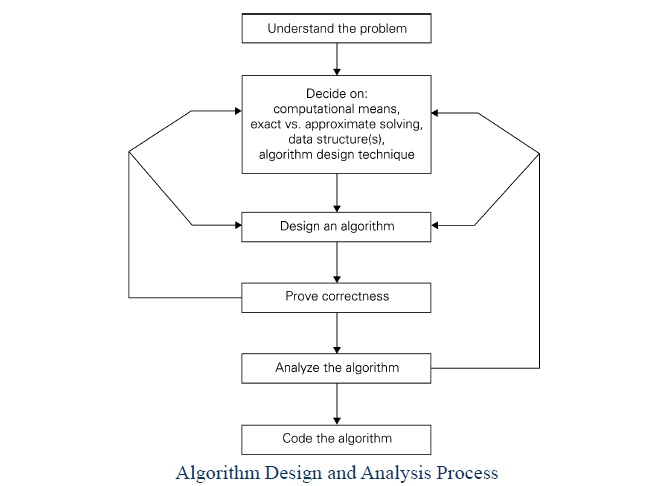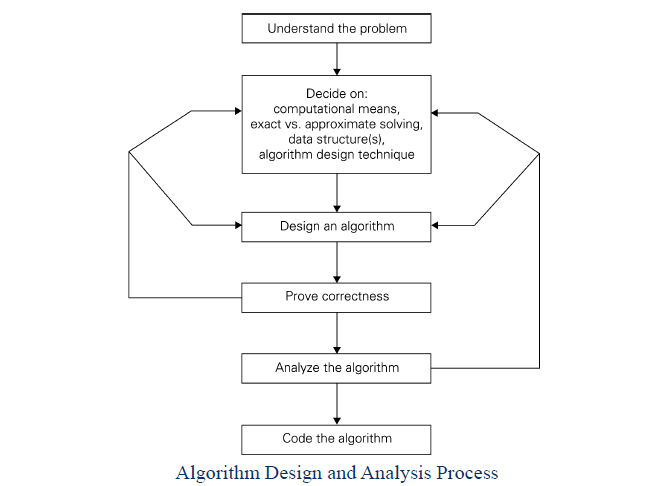# Algorithmic Problem SolvingSeptember 15, 2016

Categorised in:• Understanding the problem
• Deciding on:
• Exact vs. approximate problem solving
• Appropriate data structure
• Design of algorithm
• Proving correctness
• Analyzing an algorithm
• Time efficiency
• Space efficiency
• Coding an alogrithm